# How to Perform the SUMIF Function in Excel

How to Perform the SUMIF Function in Excel: If you are working with data, there are times when you want to take the sum of specific numbers, but looking at the numbers and tapping on the plus button on your calculator can take you hours. What if you have more than a thousand data points?

This is where the SUMIF function in Excel comes in handy. In this lesson, we will discuss how it is used, and we will help you understand what the parts are:

## What is SUMIF?

SUMIF is a formula that you use if you want to use the sum, or if you want to add, of numbers but only if these numbers met a specific condition.

What is the formula and what are the parts?

The formula for SUMIF is:

=sumif(range, criteria, [sum_range])

Always remember that:

• A formula always starts with an equal sign “=”
• It is followed by the command SUMIF
• Then an opening parenthesis
• Then the RANGE
• Followed by a comma (“,”)
• Then the CRITERIA
• Followed by a comma (“,”)
• Then the SUM RANGE
• Then a closing parenthesis.

The only three things that you will change here are the RANGE, the CRITERIA, and the SUM RANGE

• RANGE – refers to the group of cells where the formula will check if the condition is met
• CRITERIA – this is the condition that has to be met
• SUM RANGE – these are the number that the software will add, or sum, if the conditions were met

## How do we use the SUMIF?

See below an example of a data set. In the example, we have a group of people who made a sale. The problem is that there are so many people, and each person’s name appears many times. Imagine if you have a thousand rows of these names, dates, and sales.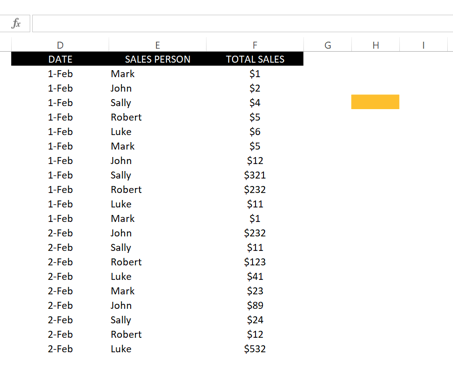Excel Sumif Function

What should you do if you only want to see the total sales of Mark? Let us say that you want to take the total sales of Mark for Feb 1 and Feb 2.

## STEP 1

Type this formula in the cell where you want the total sum to appear or in the formula box: =SUMIF(## STEP 2

Next, you have to highlight and drag the RANGE. In this case, the ranges are the cells that contain the name. Follow with a comma.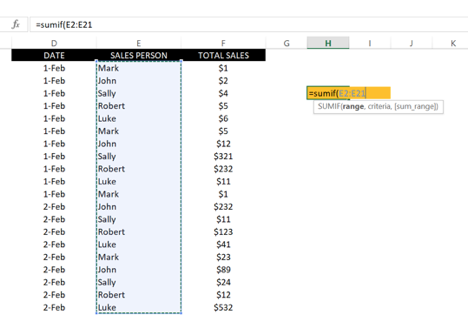As you can see, the RANGE is from E2 to E21. You are telling Excel to look in these cells.

## STEP 3

The next thing you want to do is to tell Excel the CRITERIA. In this case, the CRITERIA is Mar because you want the program to look for Mark within the RANGE of names.

To do this, type a quotation, then the name Mark, then another quotation, then a comma.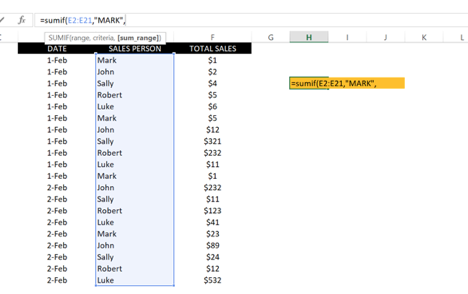The reason you need a quotation before and after Mark is that you are telling Excel that Mark is a text, not a number or a CELL.

STEP 4

The last step is to tell Excel the SUM RANGE. The SUM RANGE is the numbers that Excel will add only if it found the name Mark in your RANGE.

To do this, you need to highlight and drag cells F2 to F21 because this is where the numbers are: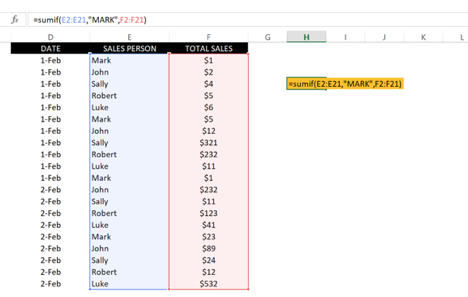Next, click Enter and you shall see the result of \$30. This is the total sales of Mark.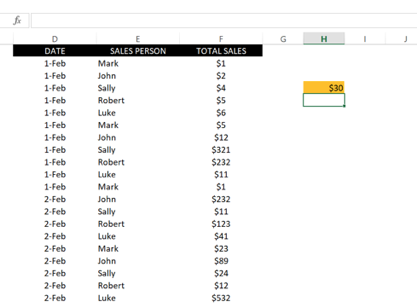Here are some pointers:

• Do not re-arrange the formula in any other way, as it will not work.
• Do not mistype the name of MARK, as the formula will not count Luke.
• Do not miss any cell in your RANGE and SUM RANGE. If you do, Excel will not count that specific cell, and you will not get an accurate number.

Now, here is another method to do this without typing the name Mark. You need to type his name somewhere and use that as a reference for the CRITERIA, instead of typing his name.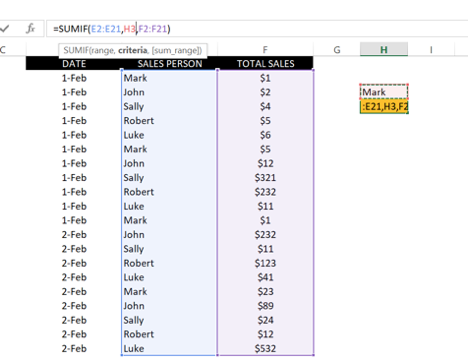As you can see, now Excel will sum every sale that is owned by the person’s name written on H3. You can replace that name with others, or you can create a table and drag the formula down so you can calculate automatically for each name.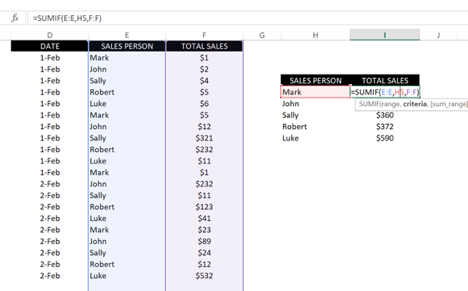Is it not awesome? Now, time for you to practice!

## How’s your data working for you?

Have you ever wonder how to blow up your business’ productivity?  You want a data expert that identifies your problems and make you look good!

If you’re still struggling to unlock all your data potential has to offer? Get in touch with us — we’d love to talk data with you.

This site uses Akismet to reduce spam. Learn how your comment data is processed.